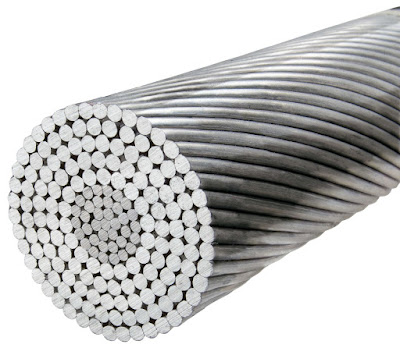# Material Selection for Transmission Line Conductor

The conductor is one of the important item in a Transmission Line and proper selection of conductor and its size is very important for economy and efficiency point of view. A conductor used for Power evacuation and distribution must have the following qualities:

• High electrical conductivity.
• High tensile strength to withstand mechanical stress.
• Low cost so that it can be used for long distance Power transmission.
• Low specific gravity so that weight per unit volume is less.

All the above mentioned qualities is generally not possessed by a single material. Therefore, while selecting conductor material for Transmission Line a compromise is made between the cost and Electrical & Mechanical properties.

The most commonly used conductor material for overhead Transmission Line are Copper, Aluminium, Steel Cored Aluminium, Galvanized Steel and Cadmium Copper.

The conductors used for overhead Transmission Line are usually stranded in order to increase the flexibility and to cope up with skin effect. In stranded conductor, there is one central wire around which layers of wires containing 6,12, 18, 24,.. wires are there.Thus if there are n layers in a Stranded Conductor, then total number of individual wire in that conductor,

= 1 + 6+ 12 +18+ 24 +…….

= 1 + 6[1 + 2 +3+ 4 +5 +……]

= 1+ 6[(n+1)n / 2] ……[Sum of n natural number = n(n+1) / 2 ]

= 1 + 3n(n+1)

Now, we will discuss the properties of each conductor material and thereby will judge their usability in overhead Transmission Line.

Copper:

Copper has dual property of high electrical conductivity and high tensile strength. Copper has high current density. Current Density is defined as the current carried by a conductor per unit cross-sectional area. Because of high current density of copper, smaller cross-sectional area of conductor is required to transmit a particular quantity of electrical power. Due to lower cross-sectional area the mechanical stress is also reduced due to wind and ice loading.

Thus we observe that Copper is an ideal material of Transmission and Distribution of electrical power. But because of its higher cost and unavailability, it is rarely used in power transmission in overhead line.

Aluminium:

Aluminium is cheap and light in weight when compared with copper but aluminium possess poor electrical conductivity and tensile strength as compared to copper. A comparison between the Aluminium and Copper is described below.

• The conductivity of Aluminium is merely 60% that of Copper. The smaller conductivity of Aluminium means that for any particular Transmission Efficiency, the cross-sectional area required for Aluminium will be more than in Copper. For the same resistance, diameter of Aluminium Conductor is around 1.26 times that of Copper Conductor.
• The specific gravity of Aluminium is lower than that of Copper. Aluminium conductor has almost one half the weight of equivalent Copper conductor. Thus supporting structure needed for Aluminium conductor need not to be much stronger.
• Due to light weight of Aluminium conductor, there is always a chance of greater swing and hence longer Cross Arm is required for Aluminium conductor.
• Because of lower tensile strength and higher coefficient of linear expansion of Aluminium, sag in Aluminium conductor will be more compared to Copper conductor.
• Considering the combined properties of conductivity, weight, tensile strength etc. Aluminium conductor is widely used for Power transmission and Distribution.

ACSR Conductor:

ACSR – Aluminium Conductor Steel Reinforced.As discussed earlier in this post, due to lower tensile strength Aluminium conductor suffers from greater sag. Thus to increase the tensile strength of Aluminium conductor, Galvanized Steel is reinforced in Aluminium conductor. The composite conductor thus obtained is popularly known as ACSR.

ACSR conductor consists of central core of Galvanized Steel strands surrounded by a number of Aluminium strands. It shall be noted that in ACSR, the cross-section of two metals are in the ratio of 1:6 which can be modified to 1:4 to achieve more tensile strength.

In ACSR composite conductor, Steel core takes the greater percentage of mechanical strength while Aluminium core carries the bulk current.

Normally, for ACSR conductor it is written as 6 / 1 or 26 / 7 ACSR. 6 / 1 ACSR means that one Galvanized Steel core is present and 6 Aluminium core surrounds the Galvanized Steel core.

Similarly, 26 / 7 ACSR means that, there are 7 Galvanized Steel cores and 26 Aluminium cores / strands surrounds them.

Galvanized Steel:

The tensile strength of Steel is very high, therefore it can be used for power transmission for long distance where the chance of mechanical loading is quite high. But because of its poor conductivity and high resistance, Galvanized Steel is not used for power transmission over a long distance. It is basically used in rural areas where cost is the main consideration.